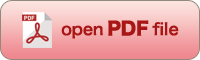[OPTICAL REVIEW Vol. 7, No. 4 (2000) 326-336]

Analysis of Off-Axial Optical Systems (2)

Keisuke ARAKI

Optics Research Dept. 31, Optics Technology Research Laboratory, Canon Inc., 3-30-2, Shimomaruko, Ohta-ku, Tokyo, 146-8501 Japan

(Received March 9, 2000; Accepted April 23, 2000)The extended 4×4 Gaussian bracket matrix Gij represents the lowest order quantity of “aberration coefficient tensor quantities” which are defined to as the peculiarity of off-axial optical systems and are independent of azimuths. We newly confirmed this extended 4×4 Gaussian bracket matrix of deflection (refraction or reflection), transmission and “twisting.” The result determined by use of a new representative method of asymmetrical surfaces and a method of paraxial expansion along the folded reference axis shows that the 4×4 Gaussian bracket matrix is the extended form of the 2×2 Gaussian bracket matrix which is used in co-axial rotational symmetric optical systems. Furthermore, we analyze and formalize the cross-term effects, which are the most serious problem in optical systems having multiple off-axial surfaces, using the concept of a chain of “optical systems divided into former and latter” and the vector-tensor analysis method. The result of this analysis reveals the structure of the cross-term effects and proves the usefulness of the vector-tensor analysis method in general image analysis.

Key words : off-axial optical system, paraxial expansion along the folded reference axis, 4×4 Gaussian bracket matrix, deflection, transmission, twisting, 4-element vectors, cross-term effects, tensor algebra, chain of “optical systems divided into former and latter”